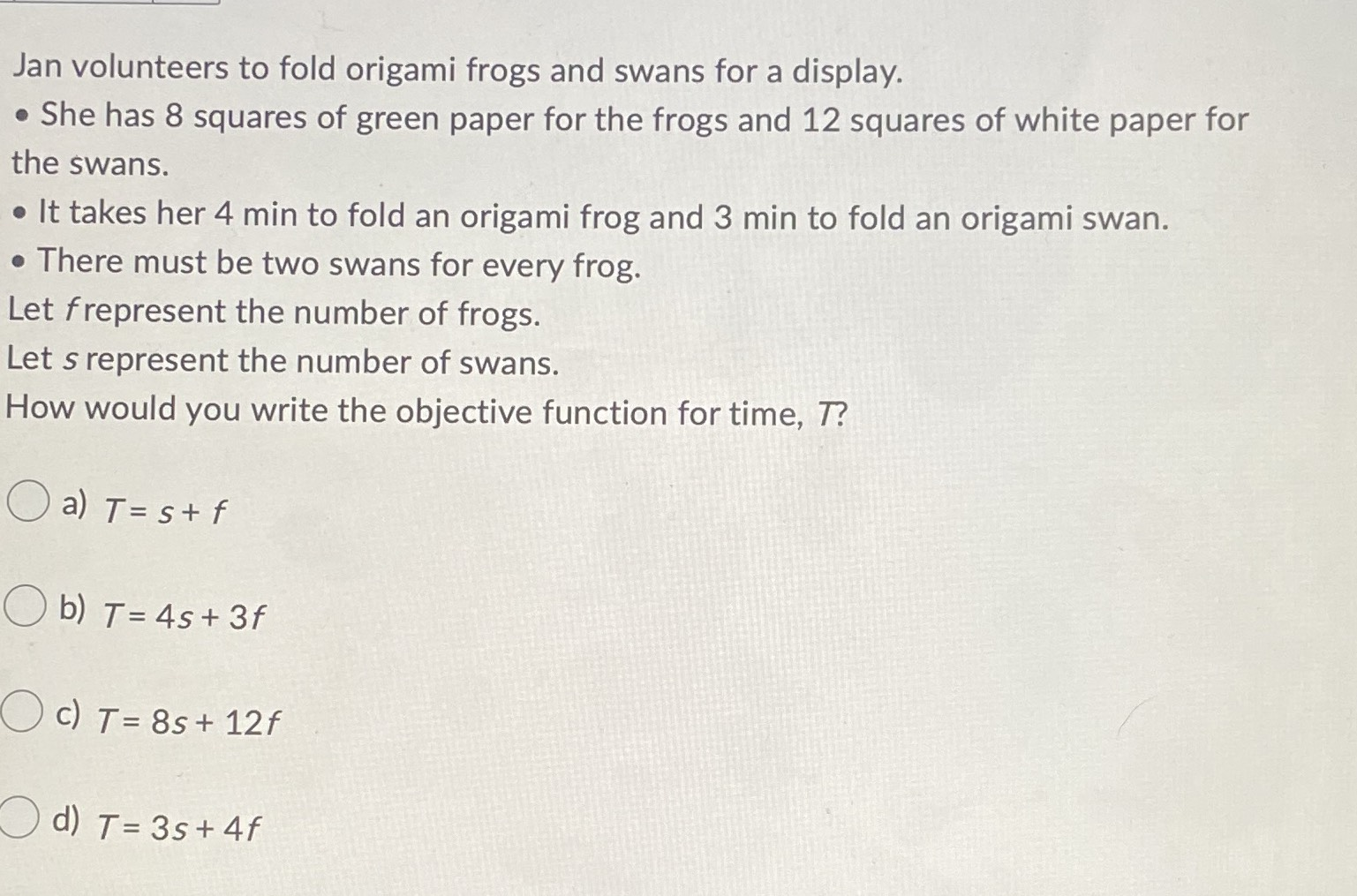### Still have math questions?

Algebra
QuestionJan volunteers to fold origami frogs and swans for a display. She has $$8$$ squares of green paper for the frogs and $$12$$ squares of white paper for the swans. - It takes her $$4$$ min to fold an origami frog and $$3$$ min to fold an origami swan. Let frepresent the number of frogs. Let s represent the number of swans. How would you write the objective function for time, $$T ?$$

a) $$T = s + f$$

b) $$T = 4 s + 3 f$$

c) $$T = 8 s + 12 f$$

d) $$T = 3 s + 4 f$$